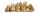# Sum of four numbers

The sum of four consecutive natural numbers is 114. Find them.

Result

a =  27
b =  28
c =  29
d =  30

#### Solution:

a+(a+1)+(a+2)+(a+3)=114

4a = 108

a = 27

Calculated by our simple equation calculator.Leave us a comment of example and its solution (i.e. if it is still somewhat unclear...):

Showing 0 comments:Be the first to comment!#### To solve this example are needed these knowledge from mathematics:

Do you have a linear equation or system of equations and looking for its solution? Or do you have quadratic equation?

## Next similar examples:

1. Write decimalsWrite in the decimal system the short and advanced form of these numbers: a) four thousand seventy-nine b) five hundred and one thousand six hundred and ten c) nine million twenty-six
2. Dropped sheetsThree consecutive sheets dropped from the book. The sum of the numbers on the pages of the dropped sheets is 273. What number has the last page of the dropped sheets?
3. Number with onesThe first digit of the number is 1, if we move this digit to the end we get a 3 times higher number, which is the number?
4. TwentyTwenty rabbits are put in 4 cells so that there are different number of rabbits in each cell contains at least 3 rabbits. What is the largest possible number of rabbits in one cell
5. Unknown number 11That number increased by three equals three times itself?
6. HotelThe hotel has a p floors each floor has i rooms from which the third are single and the others are double. Represents the number of beds in hotel.
7. GivenGiven 2x =0.125 find the value of x
8. Find xSolve: if 2(x-1)=14, then x= (solve an equation with one unknown)
9. Forest nurseryIn the forest nursery after winter, they found that 1/10 stems died out of them. For them, they land 193 new spruces. How many spruces are in the forest nursery?
10. Negative in equation2x + 3 + 7x = – 24, what is the value of x?
11. NormThree workers planted 3555 seedlings of tomatoes in one dey. First worked at the standard norm, the second planted 120 seedlings more and the third 135 seedlings more than the first worker. How many seedlings were standard norm?
12. Simple equationSolve the following simple equation: 2. (4x + 3) = 2-5. (1-x)
13. Equation 29Solve next equation: 2 ( 2x + 3 ) = 8 ( 1 - x) -5 ( x -2 )
14. Simple equationSolve for x: 3(x + 2) = x - 18
15. SimplifySimplify the following problem and express as a decimal: 5.68-[5-(2.69+5.65-3.89) /0.5]
16. AlleyAlley measured a meters. At the beginning and end are planted poplar. How many we must plant poplars to get the distance between the poplars 15 meters?
17. ExpressionSolve for a specified variable: P=a+4b+3c, for a